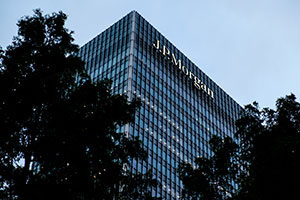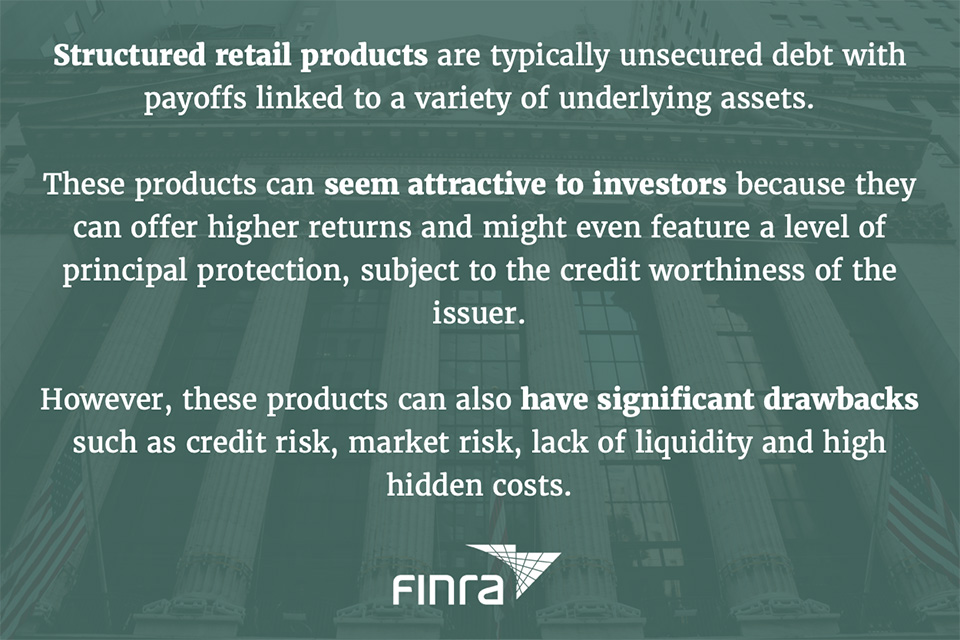# J.P. Morgan’s Callable Interest Rate Spread CDs CUSIP: 48125TBD6Did You Invest in J.P. Morgan’s Callable Interest Rate Spread CDs Linked to the 30-Year U.S. Dollar Constant Maturity Swap Rate and the 5-Year U.S. Dollar Constant Maturity Swap Rate due December 20, 2028 (CUSIP: 48125TBD6)?

According to J.P. Morgan’s prospectus on CUSIP: 48125TBD6:

The CDs are designed for investors who seek (a) periodic interest payments that (i) for the Initial Interest Periods, accrue interest at a rate equal to 10.00% per annum (corresponding to an Annual Percentage Yield (“APY”) of 10.00%) and (ii) for each Interest Period (other than the Initial Interest Periods), accrue interest at a rate equal to the Multiplier of 4.0 multiplied by the Spread, which is equal to the 30-Year CMS Rate minus the 5-Year CMS Rate minus 0.35% and (b) the return of their initial investment at maturity. For each Interest Period (other than the Initial Interest Periods), the Interest Rate is subject to the Minimum Interest Rate of 0.00% per annum (corresponding to an APY of 0.00%) and the Maximum Interest Rate of 10.00% per annum (corresponding to an APY of 10.00%).

Investors ought to be cautious with this type of investment. According to the Financial Industry Regulatory Authority (FINRA):If you bought the structured product known as J.P. Morgan’s Callable Interest Rate Spread CDs Linked to the 30-Year U.S. Dollar Constant Maturity Swap Rate and the 5-Year U.S. Dollar Constant Maturity Swap Rate due December 20, 2028 CUSIP: 48125TBD6, and it was sold to you as a safe, liquid, and/or conservative investment, and then you suffered losses, you may have a claim. Contact the attorneys at Sonn Law Group for free consultation.

Image credit: Håkan Dahlström via Flickr